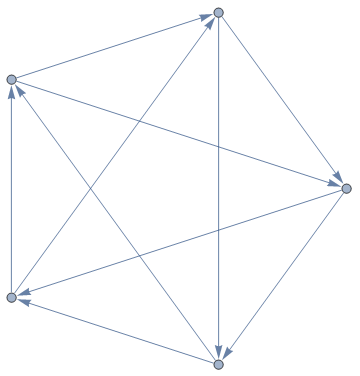# Tournament dice

In an earlier cp4space post, I presented a set of five 5-sided dice. We can draw a directed graph associated with this set of dice:Each vertex represents a die. If die A beats die B with a probability greater than ½, we draw a directed edge from A to B. If two dice have equal probability of beating each other, we leave the edge blank. We make the additional restriction that for each value x, no two dice can have x on a face; this ensures that there are no ‘draws’ between two dice.

Can we, for every directed graph, create a set of non-transitive dice corresponding to it? Radu Bumbacea answered this question in the affirmative, devising a construction for a set of n dice with 2e faces emulating any given digraph with n vertices and e directed edges. So, for the digraph given above, his construction would require five icosahedral dice. You might want to try finding a similar construction yourself.

### Tournaments, lower bounds and quadratic residues

A tournament is a complete directed graph with n vertices and$\frac{1}{2}n(n-1)$ edges. Radu’s construction gives an upper bound of$F = n(n-1)$ faces on each die. Can we establish a lower bound? Indeed, we can. There are$2^{\frac{1}{2}n(n-1)}$ different tournaments on n vertices, and$\frac{(Fn)!}{(F!)^n}$ ways to assign distinct values to n dice with F faces each. Using Stirling’s formula and considering the asymptotics, it is evident that we have a lower bound of$F ~ \frac{n - 1}{2 \log{n}}$, where the logarithm is base-2. This is considerably lower than Radu’s upper bound.

In the basic set of three non-transitive dice, we have the property that if one player chooses a die, you can choose another die so that you have a probability greater than ½ of securing a win. We can choose a more complicated tournament, where any pair of dice can be beaten by a third die. An elegant solution is to consider the dice to be integers modulo 7, and declare that one die beats another if their difference is a quadratic residue:Using Radu’s construction, we can design a set of seven 42-faced dice which beat each other according to this directed graph. It transpires that this is quite suboptimal, though, as Oskar van Deventer discovered an equivalent set of seven 3-faced dice; their double covers are shown below:The quadratic residue construction also gives a tournament with 19 vertices, where any set of three vertices is beaten by another vertex. Oskar hasn’t found an elegant set of dice for this tournament, although Radu’s construction gives a set of nineteen 342-faced dice. The order-19 tournament is rendered below, where the colour gradient indicates edge direction:It’s possible to generalise even further, constructing sets of dice such that for any subset of size k, there exists a die which beats each of them with probability ≥ ½.

This entry was posted in Uncategorized. Bookmark the permalink.

### 0 Responses to Tournament dice

1.Johnicholas says:

Hakan Kjellerstrand has a constraint programming formulation of a search for a set of nontransitive dice. It might be possible to adjust it to search for a set of dice modeling a given dag, instead of (if I understand the code correctly) a cycle.

http://www.hakank.org/minizinc/nontransitive_dice.mzn

Something like that could be used generate data points that might point to a tighter variant of Radu’s construction.

2.wojowu says:

Can you try to help me with certain combinatorical problem?
Consider finite but arbitrary number of boxes, each of which contains nonnegative integer. One of them is called counter, another one decrementor, and all other are stacks. Stacks are ordered from left to right. Let {a;b,c,…,y;z} denote counter with number a, stacks with numbers b,c,… and decrementor with number z. We define one step as changing this configuration to {a+1;b’,c’,…,y’;z’} with two constrains – sum of stacks and decrementor numbers is less than counter, and b,c,…,z is lexicographically greater than b’,c’,…,z’. Using ordinal arithmetics we can easily prove this process always terminates. A bit harder to prove is that there is maximal such mumber of steps. Let F(a,n) denote maximal such number for configuration {a;a,0,…,0;0) with n stacks. I estimated that F(a,n)~2^^…^^a with n Knuth up-arrows.
My question considers this: add one more constrain to step – b must be larger than c, c must be larger than d etc. but decrementor can still be as large as we wish. It heavily decreases growth of function. But how much exactly? Can we attain tetrational growth in terms of change of a?

•apgoucher says:

There is a maximal number of such steps by König’s tree lemma (no infinite sequence, and finitely many choices at each step). Yes, the growth rate for the original variant is certainly Ackermann-esque (c.f. coins in boxes from the first fast-growing function article). For the second problem, the variables {b,c,…,y} are limited to a number of possibilities exponential in n (and, for fixed n, polynomial in a). The value of z can double (or something like that) every time {b,c,…,y} changes, so this gives a bound of O(2^(a^n)) for the maximum final value of z.

•wojowu says:

Thank you very much. Koenig’s lemma is very strong tool, I also used it to prove that there exists such a bound. I’m a bit disappointed with this result, I hoped for at least tetrational growth possible in terms of n, but now I see why I was wrong

3.Kyle says:

I believe I have found a set that satisfies the 19-Dice problem using Oskar’s construction. I found a set of dice with 18 sides each. I would like to know how to publish this set and prove that it is minimal to the problem constraints.

•David Fowler says:

Did you end up publishing?